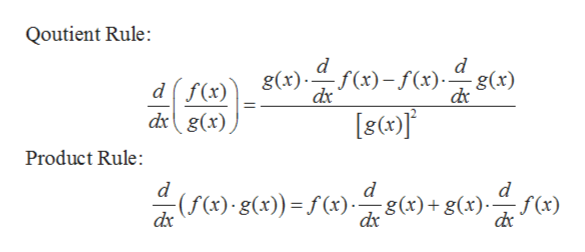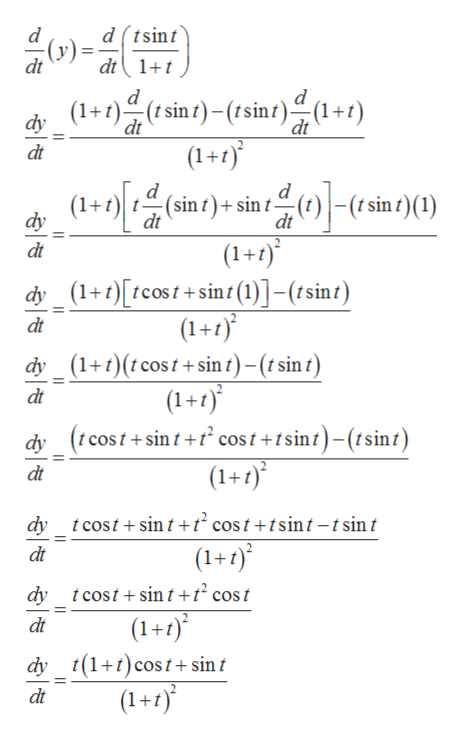2.4Can you show me the steps to solve the problem?Directions: Differentiate.13) y = (tsint)/(1 + t)

Question

2.4

Can you show me the steps to solve the problem?

Directions: Differentiate.

13) y = (tsint)/(1 + t)

Step 1

Given:

Step 2

Formulae used is given below:help_outlineImage TranscriptioncloseQoutient Rule: d d g(x) g(x)(x)(x). dx df(x) dg(x) Product Rule: d f(x) d d (f(x)g(x))=f (x)x)) dc dc fullscreen
Step 3

So now using the quotient rule and product rule differentiating th...help_outlineImage Transcriptionclosed(tsint dt1t d(y)= dt d (t sin t)- (tsint)(1+t) dy dt dt (1+1) dt d (sin t)+ sin t- dt d ()|-(1 sin t) (1) dy dt (1+1) dy(1+t)tcost+sin1(1)]-(isint) (1+1) dy (1+t)(tcost+ sin t)-(t sin t) (1+t) dy(icost+sinf+ cost+tsint)-(tsint) (1+1) dt dt dt dt tcost+sin t+t? cost +tsint-tsint dy (1+1) dt dy tcostsin t +t2 cos t (1+1) dy (1tcost+ sint (1+1) dt dt fullscreen

Want to see the full answer?

See Solution

Want to see this answer and more?

Our solutions are written by experts, many with advanced degrees, and available 24/7

See Solution
Tagged in

Derivative